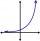# To the cinema

Jane at 8 am got message that all 1093 school pupils will go to the cinema. Within 20 min she said it to the three friends. Each of them again for 20 minutes said to the other three. In this way the message spread further. At what time all the children in school know that they will go to the cinema?

Result

x = 10:20 h#### Solution:Leave us a comment of example and its solution (i.e. if it is still somewhat unclear...):

Showing 0 comments:Be the first to comment!#### To solve this example are needed these knowledge from mathematics:

Do you have a linear equation or system of equations and looking for its solution? Or do you have quadratic equation?

## Next similar examples:

1. Insert into GPBetween numbers 5 and 640 insert as many numbers to form geometric progression so sum of the numbers you entered will be 630. How many numbers you must insert?
2. Geometric progression 2There is geometric sequence with a1=5.7 and quotient q=-2.5. Calculate a17.
3. Intercept with axisF(x)=log(x+4)-2, what is the x intercept
4. The city 3The city has 22,000 residents. How long it is expected to have 25,000 residents if the average annual population growth is 1.4%?
5. SequenceCalculate what member of the sequence specified by ? has value 86.
6. LogarithmDetermine the number whose decimal logarithm is -3.8.
7. Exponential equationIn the set R solve the equation: ?
8. Tenth memberCalculate the tenth member of geometric sequence when given: a1=1/2 and q=2
9. GP - 8 itemsDetermine the first eight members of a geometric progression if a9=512, q=2
10. Logif ?, what is b?
11. Theorem proveWe want to prove the sentense: If the natural number n is divisible by six, then n is divisible by three. From what assumption we started?
12. Five membersWrite first 5 members geometric sequence and determine whether it is increasing or decreasing: a1 = 3 q = -2
13. Powers 32 to the power of n divided by 4 to the power of -3 equal 4. What is the vaule of n?
14. Geometric sequence 4It is given geometric sequence a3 = 7 and a12 = 3. Calculate s23 (= sum of the first 23 members of the sequence).
15. Six termsFind the first six terms of the sequence a1 = -3, an = 2 * an-1
16. Exponential equationSolve for x: (4^x):0,5=2/64.
17. Geometric progressionIn geometric progression, a1 = 7, q = 5. Find the condition for n to sum first n members is: sn≤217.